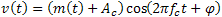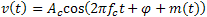# Modulation

NI RF Vector Signal Analyzers (NI-RFSA 18.1) Help

Edition Date: June 2018

Part Number: 372058U-01

View Product InfoNI-RFSA 18.1 HelpNI-RFSA 18.2.1 HelpNI-RFSA 19.1 Help

Modulation is a process that alters the characteristics of a carrier wave according to information in the message signal, m(t), to generate and transmit a modulated wave. There are several types of modulation.

The unmodulated carrier is represented by the following equation:The amplitude-modulated carrier signal is represented by the following equation:The frequency-modulated carrier signal is represented by the following equation:The phase-modulated carrier signal is represented by the following equation:where m(t) is the time-varying message signal, Ac is the amplitude of the carrier wave, and fc is the frequency of the carrier wave.

Message Signal

Carrier Wave